# 用户群组发现及兴趣用户推荐的改进的K-Means聚类算法Improved K-Means Clustering Algorithm for User Group Discovery and Interest User Recommendation

DOI: 10.12677/SEA.2019.85027, PDF, HTML, XML, 下载: 322  浏览: 737

Abstract: At present, e-commerce websites, learning resource platforms websites and social networking sites generally have the recommendation function for comment things, but not the function of users discovering and recommending users who are interested. In this paper, the FPSHK-means group discovery algorithm was designed by blending a pre-clustering stage on the basis of the K-means clustering algorithm. The pre-clustering stage includes sampling, dimensionality reduction and hierarchical clustering. The FPSHK-means group discovery algorithm is designed to recommend interested users for the users. Through the comparison experiment with the classical K-means clustering algorithm, it is verified that the FPSHK-means group discovery algorithm can find more groups than the classical K-means algorithm. And the result of clustering is closer to the actual distribution of the data object.

1. 引言

2. 相关知识和工作

2.1. K-Means聚类算法概述

K-means是一种较典型的基于样本间相似性度量的逐点修改迭代的动态聚类算法，属于机器学习中方法中的非监督学习。此算法依据设定的参数k，把n个对象划分到k个簇中，每个簇中心为簇中对象的平均值，使得每一个对象与所属簇的中心具有较高的相似度，而与不同簇的中心相似度较低。K-means算法简单易实现，并且广泛使用，其算法流程图如图1所示。

K-means聚类算法步骤描述如下：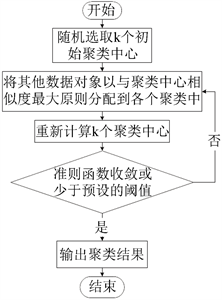Figure 1. Process of K-means clustering algorithm

K-means聚类算法  -  存在以下缺点。其一，经典的K-means聚类算法对大规模数据集的处理效率低。其二，类数目k难以事先确定，以及初始聚类中心的随机性，这两点使得K-means算法的聚类结果很多时候会陷入局部最优而难以得到全局最优。其三，因为K-means是基于样本间相似度的聚类算法，所以它只能发现球形簇。这些局限都使得经典K-means算法的聚类结果很多时候跟真实的群组分布情况相差较大。

2.2. 群组发现算法SPHK-Means概述

$\text{Precision}=\frac{|\text{true positives}|}{|\text{true positives}|+|\text{false positives}|}$ (1)

$\text{Recall}=\frac{|\text{true positives}|}{|\text{true positives}|+|\text{false negatives}|}$ (2)

$F=\text{2}\cdot \frac{\text{Precision}\cdot \text{Recall}}{\text{Precision}+\text{Recall}}$ (3)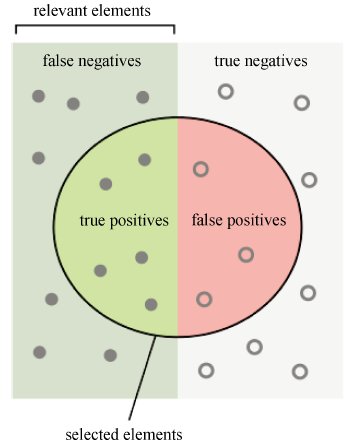Figure 2. Describe precision and recall

3. FPSHK-Means聚类算法设计

3.1. 设计思路

SPHK-means聚类算法仍有以下不足之处：

1) SPHK-means算法是在预聚类阶段的每次抽样之后对样本进行降维处理，在真聚类阶段再对数据总体降维。这样的操作顺序可能会因数据的不同而导致样本和总体的主成分分析的系数存在差异，造成样本和总体在降维时的线性变换可能略有不同，进而影响最终聚类结果。

2) SPHK-means算法是直接使用原始用户–物品稀疏评分矩阵R做聚类，对缺失值的处理方法是直接赋0值，这样会忽略用户对未知物品的潜在信息，而这些信息却能够直接影响用户聚类的结果。

3.2. 模型设计

$\stackrel{^}{R}=\left[\begin{array}{cccc}{\stackrel{^}{r}}_{1,1}& {\stackrel{^}{r}}_{1,2}& \cdots & {\stackrel{^}{r}}_{1,M}\\ {\stackrel{^}{r}}_{2,1}& \ddots & & ⋮\\ ⋮& & \ddots & ⋮\\ {\stackrel{^}{r}}_{U,1}& \cdots & \cdots & {\stackrel{^}{r}}_{U,M}\end{array}\right]=\left[{X}_{1},{X}_{2},\cdots ,{X}_{M}\right]$ (4)

FPSHK-means聚类算法的操作流程图如图3所示。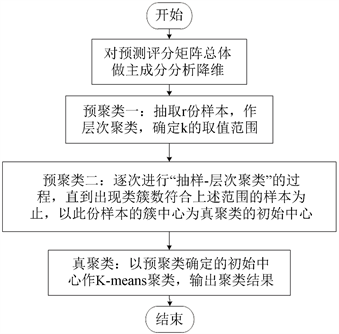Figure 3. Process of FPSHK-means clustering

FPSHK-means聚类算法步骤如下：

$\left\{\begin{array}{l}{F}_{1}={a}_{11}{X}_{1}+{a}_{21}{X}_{2}+\cdots +{a}_{M1}{X}_{M}\\ {F}_{2}={a}_{12}{X}_{1}+{a}_{22}{X}_{2}+\cdots +{a}_{M2}{X}_{M}\\ \text{\hspace{0.17em}}\text{\hspace{0.17em}}\text{\hspace{0.17em}}\text{\hspace{0.17em}}\text{\hspace{0.17em}}\text{\hspace{0.17em}}\text{\hspace{0.17em}}\text{\hspace{0.17em}}\text{\hspace{0.17em}}\text{\hspace{0.17em}}\text{\hspace{0.17em}}\text{\hspace{0.17em}}\text{\hspace{0.17em}}\text{\hspace{0.17em}}\text{\hspace{0.17em}}\text{\hspace{0.17em}}\text{\hspace{0.17em}}\text{\hspace{0.17em}}\text{\hspace{0.17em}}\text{\hspace{0.17em}}⋮\\ {F}_{p}={a}_{1p}{X}_{1}+{a}_{2p}{X}_{2}+\cdots +{a}_{Mp}{X}_{M}\end{array}$ (5)

1) 对于每个主成分 ${F}_{i}\left(i=1,2,\cdots ,\rho \right)$ 系数的平方和为1：

${a}_{1i}^{2}+{a}_{2i}^{2}+\cdots +{a}_{Mi}^{2}=1$ (6)

2) 不同的主成分 ${F}_{i}$${F}_{i}\left(i\ne j;i,j=1,2,\cdots ,\rho \right)$ 相互独立，即协方差为零：

$Cov\left({F}_{i},{F}_{j}\right)=0$ (7)

3) 所有主成分依其重要程度(方差)呈递减排列，即：

$Var\left({F}_{1}\right)\ge Var\left({F}_{2}\right)\ge \cdots \ge Var\left({F}_{p}\right)$ (8)

${R}_{red}=\left[\begin{array}{cccc}{f}_{1,1}& {f}_{1,2}& \cdots & {f}_{1,p}\\ {f}_{2,1}& \ddots & & ⋮\\ ⋮& & \ddots & ⋮\\ {f}_{U,1}& \cdots & \cdots & {f}_{U,p}\end{array}\right]$ (9)

${f}_{ui}={a}_{1i}{\stackrel{^}{r}}_{u,1}+{a}_{2i}{\stackrel{^}{r}}_{u,2}+\cdots +{a}_{Mi}{\stackrel{^}{r}}_{u,M}$ (10)

$\stackrel{¯}{k}=\frac{1}{n}\underset{i=1}{\overset{n}{\sum }}k\left(i\right)$ (11)

$\sigma \left(k\right)=\frac{1}{n}\sqrt{\underset{i=1}{\overset{n}{\sum }}{\left(k\left(i\right)-\stackrel{¯}{k}\right)}^{2}}$ (12)

4. 实验结果与分析

4.1. 实验数据集与实验环境Table 1. The top five records of movies

4.2. 评价指标

FPSHK-means算法与经典的K-means算法都属于基于相似度的聚类算法。评价这类算法通常使用误差平方和(Sum of Squared Errors, SSE)作为评价指标。

$\text{SSE}=\underset{i=1}{\overset{k}{\sum }}\underset{x\in {C}_{i}}{\sum }{\left(x-{v}_{i}\right)}^{2}$ (13)

4.3. 实验结果分析Table 2. Experimental results comparison of FPSHK-means algorithm and K-means algorithm

Segaran在他的著作中提到了用于数据可视化的多维缩放技术  ，这个是一种将多维数据集展现在二维平面上的技术。在多维缩放效果图中，数据成员两两间的距离越大，它们在原多维空间中的相似度越小。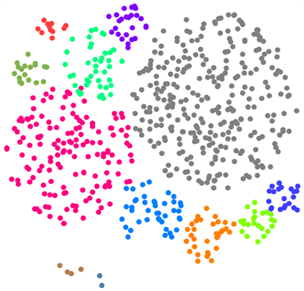Figure 4. Multidimensional scaling results of FPSHK-means clustering algorithm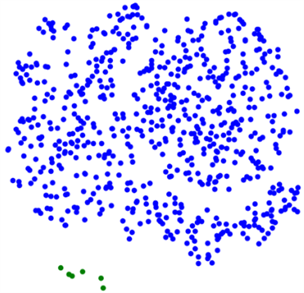Figure 5. Multidimensional scaling results of K-means clustering algorithm

5. 结语

  章永来, 周耀鉴. 聚类算法综述[J]. 计算机应用, 2019, 39(7): 1869-1882.  甘月松, 陈秀宏, 陈晓晖. 一种AP算法的改进: M-AP聚类算法[J]. 计算机科学, 2015, 42(1): 232-235+267.  王娟. 一种基于遗传算法的K-means聚类算法[J]. 微型机与应用, 2011, 30(20): 71-76.  Arthur, D. and Vassilvitskii, S. (2007) K-Means++: The Advantages of Careful Seeding. In: Proceedings of the 18th Annual ACM-SIAM Symposium on Discrete Algorithms, Society for Industrial and Applied Mathematics, Philadelphia, PA, 1027-1035.  Goel, L., Jain, N. and Srivastava, S. (2016) A Novel PSO Based Algorithm to Find Initial Seeds for the K-Means Clustering Algorithm. Communication and Computing Systems Clustering Algorithm. 2016 The International Conference on Communication and Computing Systems, Gurgaon, 9-11 September 2016, 159-163.  Gu, L. (2017) A Novel Locality Sensitive K-Means Clustering Algorithm Based on Subtractive Clustering. 2016 7th IEEE International Conference on Software Engineering and Service Science, Beijing, 26-28 August 2016, 836-839. https://doi.org/10.1109/ICSESS.2016.7883196  谢娟英, 王艳娥. 最小方差优化初始聚类中心的K-Means算法[J]. 计算机工程, 2014, 40(8): 205-211+223.  贾瑞玉, 李玉功. 类簇数目和初始中心点自确定的K-Means算法[J]. 计算机工程与应用, 2018, 54(7): 152-158.  马福民, 逯瑞强, 张腾飞. 基于局部密度自适应度量的粗糙K-Means聚类算法[J]. 计算机工程与科学, 2018, 40(1): 184-190.  丛思安, 王星星. K-Means算法研究综述[J]. 电子技术与软件工程, 2018(17): 155-156.  明小红. 基于用户聚类的协同过滤推荐算法研究[D]: [硕士学位论文]. 北京: 北京交通大学, 2017.  王千, 王成, 冯振元, 叶金凤. K-Means聚类算法研究综述[J]. 电子设计工程, 2012, 20(7): 21-24.  Li, D.-Y. and Cao, C.-F. (2017) An Improved K-Means Clustering Algorithm Applicable to Massive High-Dimensional Matrix Datasets. Proceedings of the 2017 International Conference on Information Science and Technology, EDP Sciences, Wuhan, 24-26 March 2017, 269-275.  Li, D.-Y. and Cao, C.-F. (2017) Discovering Movie Categories Based on SPHK-Means Clustering Algorithm. Proceedings of the 2017 International Conference on Information Science and Technology, EDP Sciences, Wuhan, 24-26 March 2017, 276-283.  https://en.wikipedia.org/wiki/Precision_and_recall  Shlens, J. (2014) A Tutorial on Principal Component Analysis. https://arxiv.org/pdf/1404.1100.pdf  Segaran, T. (2015) Programming Collective Intelligence. Publishing House of Electronics Industry, Beijing, 49-52.